What is the Intuition Behind Machine Learning?

# What is the Intuition Behind Machine Learning?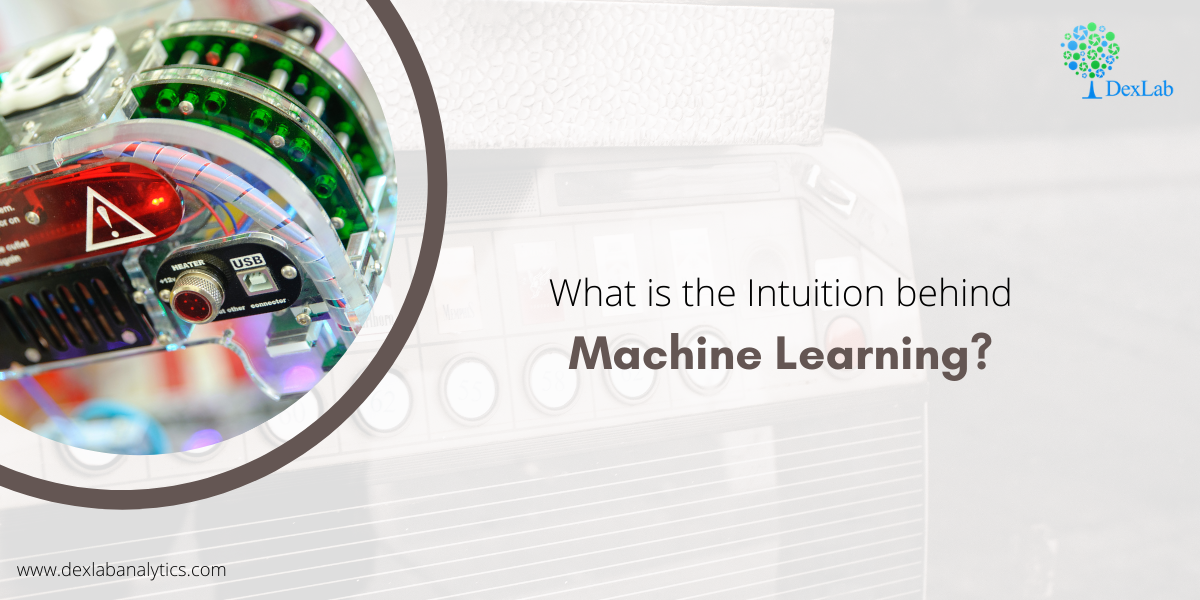Machine learning has become a popular term as this advanced technology is full of immense potential. Before explaining the intuition behind machine learning let’s understand the meaning of the term first which is becoming so popular in this era of scientific innovation and is a trend that everybody wants to follow.

#### What is Machine Learning?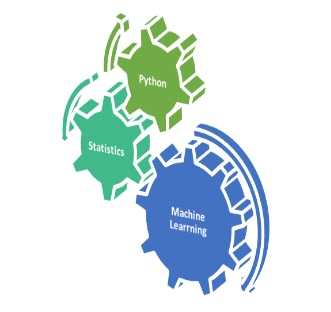Machine learning if explained in a very layman language is a program running behind an application which has an ability to learn from what is sees and the errors that it makes and then tries to improve itself through trial and error. A programming language like Python and a method of calculation (statistics) is what helps propel this application in the right direction.

Now that you know what machine learning is, let’s discuss about what is the intuition behind building a machine learning algorithm or a program.

In my previous blog I have discussed about a statistical concept called Linear Regression which follows given a X independent variable, prediction of a Y dependent variable is possible if we understand the rate at which X and Y are changing and the direction towards which they are moving i.e. we understand the hidden pattern they are following, we will  be able to predict the value of Y when X= 15.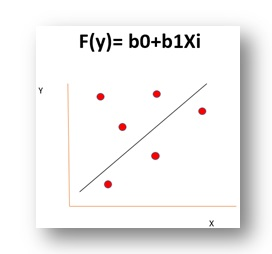In the process of all that, we need to reduce the error between the predicted Y and the observed Y which we had to train our model but this is not possible with only calculating the slope i.e. b1 a single time and this is where machine learning comes in handy.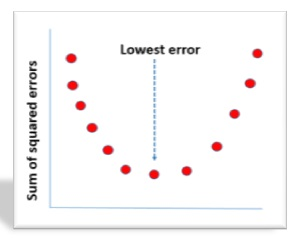The idea behind machine learning is to learn from the past mistakes and try to find the best possible coefficients i.e. b0 and b1 so that we are able to reduce the distance between predicted and observed y which leads to the minimization of error in predictions which we are making. This intuition remains the same throughout all the machine learning algorithms only the problem in question and the methodology to solve the problem changes.

Now let’s quickly look at the branches of Machine Learning.

#### Branches of Machine Learning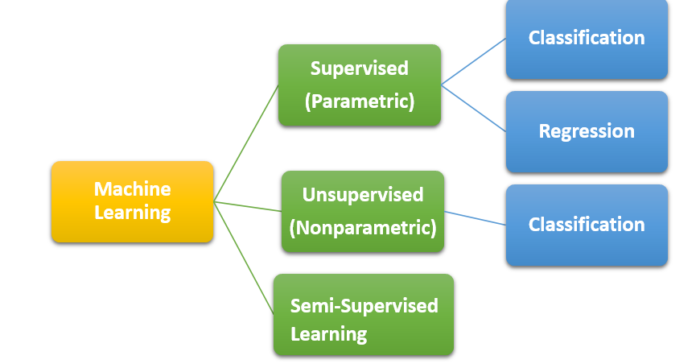• Supervised (Parametric) Machine Learning Algorithm:- Under this branch both the independent variable X and the dependent variable Y is given in the form of Y = f(X) and this branch can further be divided based on the kind of problem we are dealing with i.e. whether the variable Y is continuous or a category.
• Unsupervised (Non-parametric) Machine Learning Algorithm:- Under this branch you do not have the Y variable i.e. Y ≠ f(X) and you can only solve classification problems.
• Semi-Supervised Machine Learning Algorithms:- This is the most difficult to solve as under this kind of problem the data which is available for the analysis has missing values of Y which makes it quite difficult to train the algorithm as the possibility of false prediction is very high.

So, with that this discussion here on machine learning wraps up,  hopefully, it helped you understand the intuition behind machine learning, also check out the video tutorial attached down the blog to learn more. The field of machine learning is full of opportunities, DexLab Analytics offers machine learning course in delhi ncr, keep on following the blog to enhance your knowledge as we continue to update it with interesting and informative posts for you.

.

+91 931 572 5902